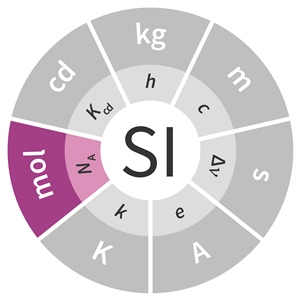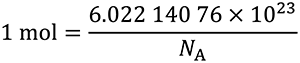# SI base unit: mole (mol)

The mole, symbol mol, is the SI unit of amount of substance. One mole contains exactly 6.022 140 76 x 1023 elementary entities. This number is the fixed numerical value of the Avogadro constant, NA, when expressed in the unit mol–1 and is called the Avogadro number.

The amount of substance, symbol n, of a system is a measure of the number of specified elementary entities. An elementary entity may be an atom, a molecule, an ion, an electron, any other particle or specified group of particles.This definition implies the exact relation NA = 6.022 140 76 x 1023 mol–1. Inverting this relation gives an exact expression for the mole in terms of the defining constant NA:The effect of this definition is that the mole is the amount of substance of a system that contains 6.022 140 76 x 1023 specified elementary entities.